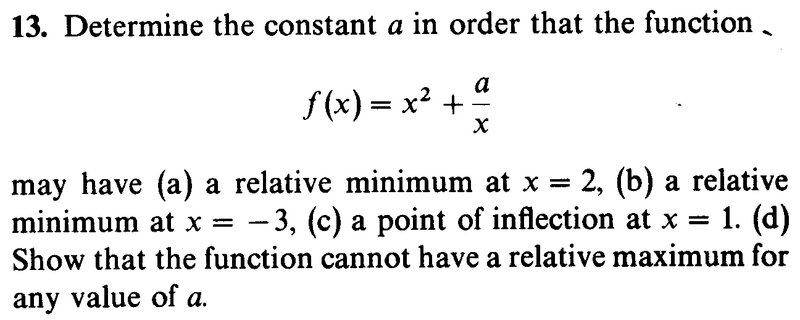# Local minimum and maximum

Karol

## Homework Statement## Homework Equations

At local minimum or maximum the first derivative equals zero

## The Attempt at a Solution

a) $$f'=2x-\frac{a}{x^2},~~2\cdot 2-\frac{a}{4}=0~\rightarrow a=16$$
Near 0 from the left a/x gets large negative values, smaller than for a=16. that's my proof for the local minimum.
The same for b).
But how to prove c)?
If a>0, f'<0 for x<0. so f decreases. for x>0 we found a minimum, and f''>0, so it holds water.
Similar reasoning for a<0. is this the way?

Mentor

## Homework Statement

View attachment 215032

## Homework Equations

At local minimum or maximum the first derivative equals zero

## The Attempt at a Solution

a) $$f'=2x-\frac{a}{x^2},~~2\cdot 2-\frac{a}{4}=0~\rightarrow a=16$$
Near 0 from the left a/x gets large negative values, smaller than for a=16. that's my proof for the local minimum.
The same for b).
But how to prove c)?
If a>0, f'<0 for x<0. so f decreases. for x>0 we found a minimum, and f''>0, so it holds water.
Similar reasoning for a<0. is this the way?
Part c doesn't ask you to prove anything -- just find the inflection point.
Part d is the "show" part. If there were a rel. maximum somewhere, what must be true?

Karol
If there were a rel. maximum somewhere, what must be true?
Then f'=0 and f''<0 there, and there would have been higher points.
The end points at infinity, both for x>0 and x<0 are higher.
$$f'=2x-\frac{a}{x^2},~~f'=0~\rightarrow x=\sqrt{\frac{a}{2}}$$
$$f''=2x-\frac{1}{x^2},~~f''\left( \sqrt{\frac{a}{2}} \right)=...=\sqrt{\frac{4}{a^2}}(a-1)$$
For a>1 f''>0 at ##~\displaystyle x=\sqrt{\frac{a}{2}} so it's a local minimum.
But for 0<a<1 f''<0

Mentor
Then f'=0 and f''<0 there, and there would have been higher points.
The end points at infinity, both for x>0 and x<0 are higher.
$$f'=2x-\frac{a}{x^2},~~f'=0~\rightarrow x=\sqrt{\frac{a}{2}}$$
I almost agree with the above.
Suppose there is a relative maximum at x = c.
Then ##f'(c) = 2c - \frac a {c^2} = 0## and ##f''(c) < 0##
Solving for c in the first equation gives ##c =\sqrt{\frac a 2}##, the same value you show.

Karol said:
$$f''=2x-\frac{1}{x^2},~~f''\left( \sqrt{\frac{a}{2}} \right)=...=\sqrt{\frac{4}{a^2}}(a-1)$$
This part (above) is incorrect. ##f''(x) \ne 2x - \frac 1 {x^2}##
Redo your calculation for f''(x), and then evaluate f''(c) and see whether f''(c) can ever be negative.
Karol said:
For a>1 f''>0 at ##~\displaystyle x=\sqrt{\frac{a}{2}} so it's a local minimum.
But for 0<a<1 f''<0

Karol
$$f''=2x-\frac{1}{x^3},~~f''\left( \sqrt{\frac{a}{2}} \right)=...=6$$
So for every a, f holds water

Mentor
$$f''=2x-\frac{1}{x^3},~~f''\left( \sqrt{\frac{a}{2}} \right)=...=6$$
So for every a, f holds water
##f''(\sqrt{\frac a 2}) \ne 6##, so your conclusion doesn't hold water. Also, if it were true that ##f''(\sqrt{\frac a 2}) = 6## (which isn't true), then that would mean that the graph of f would hold water.

Karol
$$f''=2x-\frac{1}{x^3},~~f''\left( \sqrt{\frac{a}{2}} \right)=2\cdot \sqrt{\frac{a}{2}}-\frac{2}{a}=2\left[ \sqrt{\frac{a}{2}}-\frac{1}{a} \right]$$

Homework Helper
Gold Member
2022 Award
$$f''=2x-\frac{1}{x^3},~~f''\left( \sqrt{\frac{a}{2}} \right)=2\cdot \sqrt{\frac{a}{2}}-\frac{2}{a}=2\left[ \sqrt{\frac{a}{2}}-\frac{1}{a} \right]$$
The problem is not that complicated. In part a) you supposed the function had a local extremum. How many values of a did you find?

Mentor
$$f''=2x-\frac{1}{x^3},~~f''\left( \sqrt{\frac{a}{2}} \right)=2\cdot \sqrt{\frac{a}{2}}-\frac{2}{a}=2\left[ \sqrt{\frac{a}{2}}-\frac{1}{a} \right]$$
Your work is incorrect right from the start. For one thing, you lost the factor of a that was present in the original function.
##f(x) = x^2 + ax^{-1}##
##f'(x) = 2x - ax^{-2}##
##f''(x) = ?##
If f has a relative maximum, f''(a) must be positive for some value of a.

Karol
$$f''=2\left[ 1+\frac{a}{x^3} \right]$$
$$f''\left( \sqrt{\frac{a}{2}} \right)=6$$

Mentor
$$f''=2\left[ 1+\frac{a}{x^3} \right]$$
This is f''(x)
Karol said:
$$f''\left( \sqrt{\frac{a}{2}} \right)=6$$
So what do you conclude?